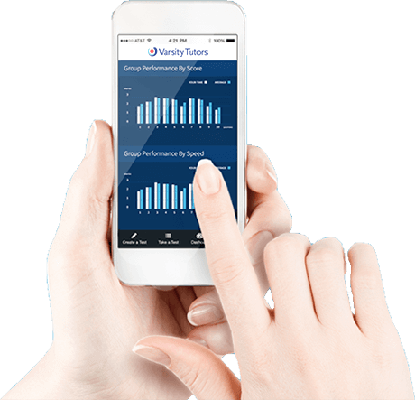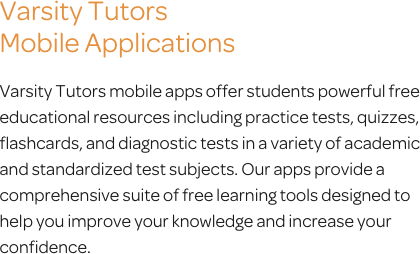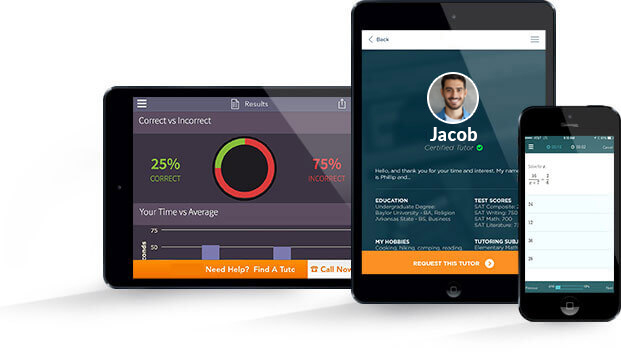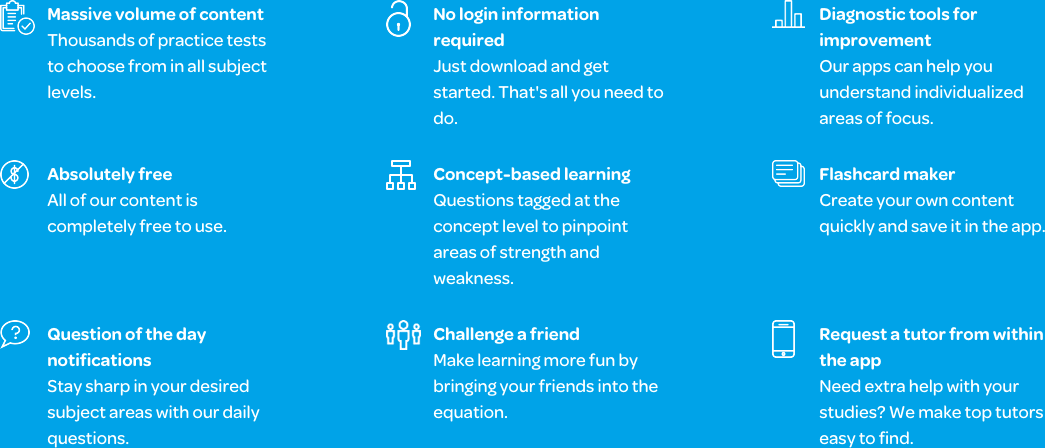# Varsity Tutors Pre-Calculus mobile app### The Varsity Tutors Pre-Calculus Mobile App

If you are a high school or college student taking a pre-calculus course, you could benefit from getting some extra assistance to help you make sense of the material. Despite being an important component of a number of fields, from engineering to computer science, to even medicine, pre-calculus can be challenging for many students who struggle with mathematics. Fortunately, Varsity Tutors’ Learning Tools can help you get the assistance you need to succeed on any upcoming exams.

If you have an Android or iOS device, you should consider downloading the Varsity Tutors Pre-calculus mobile learning app. This app is free of charge, and it has comprehensive study material that can help you get a better understanding of the course. Here, you are a push of a button away from reviewing circles, ellipses, hyperbolas, parabolas, and a number of other common themes in the course. Whether you need to learn concepts from the beginning, or you simply want to supplement your review sessions, this program can help you get the assistance needed to succeed.

Consider using the Question of the Day as a way to get a quick study session. Here, you will be asked a different question each day about something central to pre-calculus. One day you might be given a problem on quadratic systems, and the next day you could be asked something about growth and decay of exponential functions. It is a great way to begin a study session or to simply get quick practice every day.

If you want to learn comprehensively, access the Learn by Concept feature. This is an interactive syllabus that has all of the course content laid out, so you can choose which sections you want to learn about. Consider it like an electronic study guide that covers everything from logarithmic functions, the Cartesian coordinate systems, and real numbers to graphic functions. Anything that is covered over the duration of the course should be present in this portion of the study program.

Once you have reviewed, you can reinforce your knowledge with the flashcards. There are over a thousand cards available, and they are broken down by concepts. This means that you can review a wide range of subjects, or you can focus on a specific area like quadratic equations, harmonic motion, the area under a curve, or derivatives.

You can also test your knowledge through the practice tests. If you want to get a good idea of how you will do on test day, try taking the Full-Length Practice Tests. These tests are long and comprehensive, and they are designed to emulate an actual exam. If you want to evaluate specific areas, like matrices and vectors, inductor calculus, or polynomial functions, consider taking one of the short-length practice tests. You can spend a few minutes reviewing a number of specific areas, as well as short-length general tests. Whichever test you choose, you can check your answers and read detailed explanations about how each correct answer was reached. You can also look at various statistics, like how long it took to answer each question and where you rank against other test-takers.

No matter what your proficiency level or actual goals are, the Varsity Tutors Pre-calculus app can help you get the assistance you need. It is available on iTunes and the Google Play Store and is a perfect addition to your study regimen.Precalculus is a college-level course that you may take during high school. If you take advanced math classes, you can be better prepared prior to college, ensuring that you can excel in the more rigorous math concepts presented there. With precalculus, you receive a combination of trigonometry and college algebra as a form of preparation for calculus.

Considering the wide range of concepts to become familiar with, you may be wondering what you will face in the coming school year. The free Precalculus app by Varsity Tutors shows you everything you need to know, and you can download it from iTunes and the Google Play Store for your iPhone, iPad, or Android-powered phone or tablet. From conic sections to logarithmic functions, you will need to be capable of working with graphs, shapes, and equations in detail. There are numerous important functions that you must know in order to move on to calculus coursework, such as basic algebraic functions and the Cartesian coordinate system, which is vital to graphing certain equations. You must be capable with trigonometric identities, capable of identifying a decimal value in trigonometric equations, and solving special angles using specific equations.

Your precalculus course begins with conic sections and ends with trigonometric identities. You need to be capable of differentiating between the four types of conic sections: parabola, circle, hyperbola, and ellipse. You will need to learn how to graph circles correctly, as well as how you can identify the center, foci, eccentricity, and endpoints of an ellipse. You will learn about solving the equation of a hyperbola using the standard form before graphing it. You’ll work with parabolas, learning how to determine the direction it will open based on the equation. In addition, you’ll learn to solve for the polar equation of a given conic, identify a conic with a given polar equation, and solve quadratic equations using the appropriate techniques.

Exponential functions are very different from logarithmic ones. You need to be capable of differing between them. You can use these numerous formulas to solve for a wide range of problems, such as exponential growth and decay. Furthermore, these can be applied to expressions, allowing you to simplify and evaluate rational exponents with ease.

You will need to be familiar with the wide number of functions that you will use in precalculus, all of which are covered in Varsity Tutors’ free Precalculus app for iPhone, iPad, and Android. These functions are vital to your ability to compose new functions. You will learn how to perform basic operations with functions, such as multiplication and addition. Further, you’ll need to develop an understanding of the Cartesian coordinate system and how it relates to graphing equations or plot points respectively. Throughout this section of your class, you’ll face exercises designed to help you learn to use linear modeling to demonstrate your answers, as well as methods that allow you to find the inverse of a function and a relation. You’ll be tasked with developing the skills necessary to identify a relation that is a function, as well as to evaluate various functions.

Graphing is a critical component to precalculus. During your precalculus class, you will develop a number of skills to ensure that you master the various graphing strategies. You will start by focusing on determining the distance between parallel lines, points, and lines. In addition, you’ll learn how to transform a quadratic function into a vertex. You must become proficient in graphing quadratic functions by identifying specific characteristics. Linear functions, polynomial equations, and quadratic equations take up a significant portion of your graphing-focused coursework.

When your teacher begins to introduce tangent, secant, cosecant, cotangent, sines, and cosines, you will be learning how to take these functions and use them to solve various equations. Armed with a graphing calculator, you may be tasked with determining the amplitude of a cosine or sine function, phase shifts in a similar function, or graphing it onto your paper. Further, you’ll learn how harmonic motion works, which incorporates real-world applications once you have a thorough understanding of these concepts.

From there, you’ll begin delving deeper into inequalities, quadratics, and equations. You will need to learn how to solve inequalities for their absolute value, as well as how to graph linear inequalities correctly. You’ll spend time learning to solve a variety of linear and nonlinear systems of equations, graph rational and polynomial inequalities, and solve for a quadratic inequality.

Introductory calculus focuses on the concepts necessary to lay the foundation necessary for calculus. You will learn how to use limits and rectangles to find areas, strategies to identify equations and slopes in line tangents, and determine the limits of a function. Furthermore, you’ll get an introduction to working with derivatives, which can allow you to determine inflection points, solve rate of change problems, and work with maximum problems, which require you identify the maximum and minimum of a function.

Next, you will start working with matrices and vectors. You must be able to differentiate from the two, as well as be able to find the sum and difference between two given matrices. You’ll learn to express vectors in various formats, as well as how to use two points to find the vector equation with ease. Furthermore, you will learn how to solve systems of equations with the use of augmented matrices, which require a unique formula and strategy. Finally, you’ll spend time learning how to determine parallel and perpendicular vectors, as well as the measurements between them. Just like every other topic in the course, you can practice vectors in-depth when you download the Varsity Tutors Precalculus app to your iPhone, iPad, or Android device.

From vectors, you progress into complex numbers and polar coordinates. You’ll learn how to take polar coordinates and equations and convert them into rectangular coordinates and forms. You must know the strategies required to express complex numbers in various formats, as well as to use DeMoivre’s Theorem to evaluate the power of complex numbers. Polynomials follow closely behind, where you must become familiar with various pertinent rules, such as Descartes’ Rule and the Intermediate Value Theorem. You must be able to work with rational and radical functions, which may include graphing, solving, and interpreting them. Furthermore, you’ll learn how sequences and series can play an important role in terms, sums, notation, and arithmetic.

When you delve into trigonometry, you will begin learning the very important basics to your future coursework. There are many applications you must become familiar with in order to succeed. You will begin by learning to find the area of a triangle, which may involve using Heron’s formula or using the hypotenuse. You’ll spend time working with the law of cosines and sines before you begin learning to solve right and isosceles triangles. From there, you will spend time learning how to measure and convert radians and degrees using various strategies. In addition, you must learn how to solve for angles in a coordinate plane, velocity problems, and circular functions.

Finally, you will spend precalculus studying trigonometric identities. There are numerous types of identities, which you need to be able to identify, prove, and evaluate. You may be tasked with expressing product and sum identities or solving inequalities using quadratic formulas.

Precalculus contains a wealth of course material for you to wade through. It seems like a lot, but you can work to tie it all together with Varsity Tutors’ free Precalculus app. Download it today from iTunes or the Google Play Store.